# Understanding Ratios with Examples and Pictures

Understanding Ratios with Examples and Pictures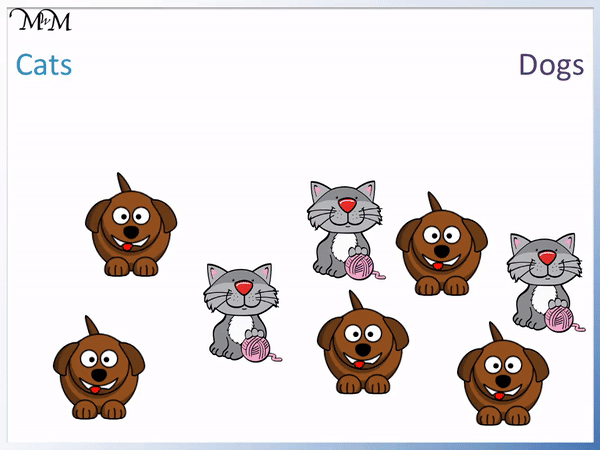• A ratio tells us how many of one object there are compared to another.
• There are 3 cats and 5 dogs, which makes 8 animals in total.
• We say there are 3 cats to every 5 dogs in this picture.
• We write the numbers of each object as a ratio, separated by a colon ‘:’.
• The ratio of cats to dogs is 3:5.
• We read this as ‘3 to 5’
• It is important to write the numbers in the same order in which they are mentioned.
• Writing the ratio the other way around, the ratio of dogs to cats is 5:3.
We write the number of each object separated by a colon sign ‘:’. .• We count the number of each coloured counter.
• We have 3 blue counters.
• We have 2 purple counters.
• We say that we have 3 blue to 2 purple.
• We write the ratio of blue to purple as 3:2.Supporting Lessons# Introduction to Ratios

## What is a Ratio?

In simple terms, a ratio is the amount of one item compared to the amount of another. Ratios describe how much of an item there is for a given amount of the other.

Ratios are commonly used to help describe the number of items in a collection.

For example here is a collection of pets. We have both cats and dogs.There are 8 animals in total.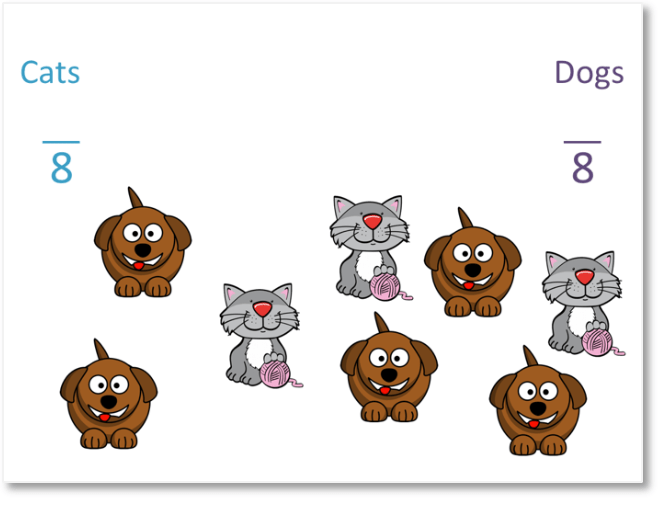There are 3 cats. 3 out of the 8 animals are cats.

We can say that   3 / 8   of the pets are cats.There are 5 cats. 5 out of the 8 animals are dogs.

We can say that   5 / 8   of the pets are dogs.

It is easier to compare amounts by writing them as a ratio than as fractions. This is because ratios simply tell us how much of each thing there is compared to another.

We have 3 cats, so we write a 3.We have 5 dogs, so we write a 5 next to the 3.

In between the 3 and the 5 we put a colon.

Colons are used to show that the numbers are written as part of a ratio. It is important to separate each number in the ratio with a new colon. The colon in a ratio is simply read as ‘to’. For example, the ratio 3:5 is read as ‘three to five’.

There are 3 cats to 5 dogs.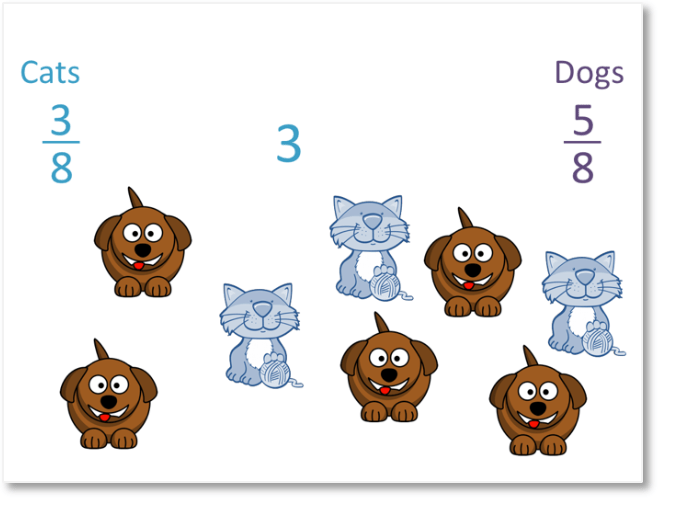Order matters when writing ratios. The first number tells us how much of the first item described there is. The second number tells us how much of the second item described there is. We cannot put the numbers in a ratio in any order.

In this example, we say that the ratio of cats to dogs is 3:5.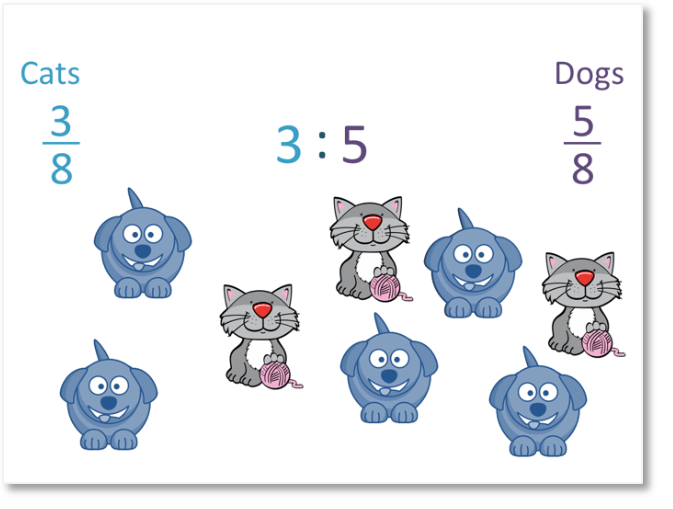Instead of the ratio of cats to dogs we were asked for the ratio of dogs to cats, we would have to reverse the ratio to say 5:3.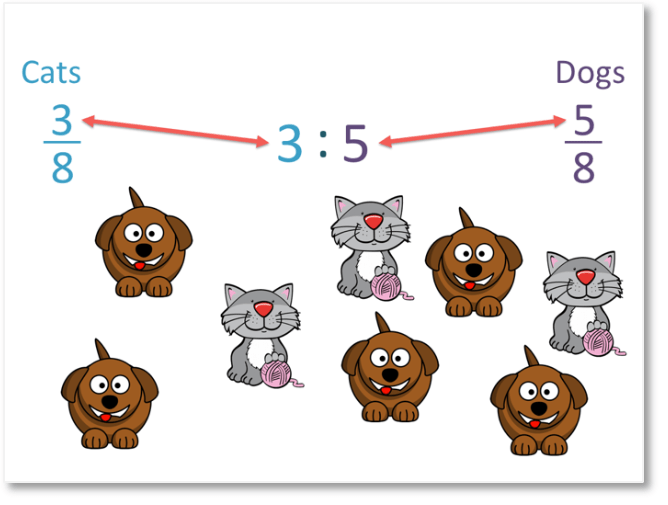Here is another example of writing a ratio.

We have 6 toy bears to 4 toy cars.We say that the ratio of bears to cars is 6:4.

Since both 6 and 4 are both even, we can simplify the ratio by halving both numbers.

We can halve 6:4 to make 3:2.

We can also say that the ratio of bears to cars is 3:2. This means that for every 3 bears there are, there are 2 cars. Every time I add 3 bears, I add 2 cars.

## Examples of Where Ratios are Written in Real Life

Examples of where ratios are written in real life include:

• Ingredients in recipes
• Making drinks
• Food prices given in price per weight
• Calculating the time taken to reach a destination
• Voting
• Speed given as distance per hour in a car

## Why do we Use Ratios?

Ratios are used to compare the amount of something compared to the amount of something else. They are paticularly useful for comparing large amounts in simple terms or for scaling up calculations. For example if you have enough ingredients for one cake, you can then work out how many of each ingredient you need to make ten cakes by using ratios.

Ingredients in recipes often contain ratios. For example, when baking a cake we might have a ratio of:

200g butter : 4 eggs : 180g flour : 1 spoon of baking powder : 3 spoons of milk.

This ratio tells us how much of each ingredient we need compared to the other. For example, if I put the ingredients above into a cake but instead of 3 spoons of milk I used 5 spoons, then the mixture might be too wet from adding too much milk.

Ratios also tell us how to scale up amounts. For example if I wanted to make 10 cakes, I could take the ingredients for 1 cake and multiply them by 10.

I would then need the following ingredients for 10 cakes:

2000g butter : 40 eggs : 1800g flour : 10 spoons of baking powder : 30 spoons of milk.

This helps me work out how much I need and I can buy the correct quantities at the shop.Now try our lesson on Introduction to Sharing in a Ratio where we learn how to divide an amount in a given ratio by sharing parts.